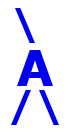## AIRES library reference: spinjpoint.

```c
c     Routine directly callable from C/C++ (it could be necessary to
c     append an underscore, "_", to its name depending on the C/C++
c     compiler used).
c
c
subroutine spinjpoint(csys, x0, y0, z0, tsw, t0beta, irc)
c
c     Setting the current injection point for primary particles.
c
c     Written by: S. J. Sciutto, La Plata 1999, 2003, 2010, 2019.
c
c
c     Arguments:
c     =========
c
c     csys............ (input, integer) Parameter labelling the
c                      coordinate system used. 0 = AIRES coordinate
c                      system. 1 = Injection point-shower axis system.
c     x0, y0, z0...... (input, double precision) Coordinates of the
c                      injection point with respect to the chosen
c                      system (in meters).
c     tsw............. (input, integer) Injection time switch. If
c                      tsw is zero then t0beta is an absolute injection
c                      time; if tsw is 1, then the injection time is
c                      set as the time employed by a particle whose
c                      speed is t0beta * c to go from the original
c                      injection point to the intersection point of
c                      the shower axis with the plane orthogonal to
c                      that axis and containing the point (x0, y0, z0).
c     t0beta.......... (input, double precision) The meaning of this
c                      argument depends on the current value of "tsw".
c                      It can be the absolute injection time (ns)
c                      (time at original injection is taken as zero);
c                      or the relative speed of a particle with
c                      respect to c.
c     irc............. (output, integer) Return code. 0 means normal
c                      return.
c
c
c<--->
```

 These pages are maintained by Sergio J. Sciutto Back to AIRES library index Back to AIRES home